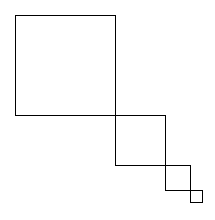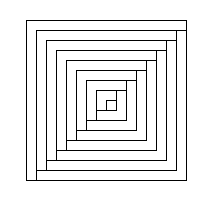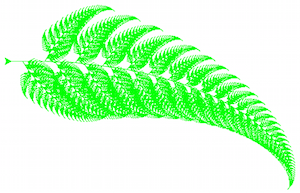# CS101 - Homework 5

Due: 2018-03-21 11:59p

#### Objectives

• Gain more practice with recursion
• Gain practice with iteration (for loops and while loops)
• Gain practice with lists

### [10 points] Prelab

Before coming to lab on Thursday / Friday, read this entire homework write-up and complete the prelab.

### [10 points] StairsUsing turtle graphics, write a recursive function ```drawStairs(len, levels)``` to draw pictures such as the one to the right. Assume the turtle starts in the top left corner, facing right. The first square should have a side length of "len", and each subsequent square should have 1/2 the size. There should be a total of "levels" squares. Do this all in one continuous drawing motion; Do not pick up the pen, and do not repeat any lines. Employ the following strategy: (1) draw a half-square, (2) recursively draw the rest of the squares, (3) draw the last half-square to return to the starting position and orientation. For testing, feel free to use the following code.

``````def drawStairsScene():
"""
Moves the turtle to the top left of the screen and draws a staircase.
"""
# pick up the pen and move the turtle so it starts at the left edge of the canvas
turtle.penup()
turtle.goto(-turtle.window_width()/2 + 20, turtle.window_height()/2 - 20)
turtle.pendown()

# draw the tree by calling your function
drawStairs(200,5)

# finished
turtle.done()
``````

### [5 points] Testing for primality

Write a function `isPrime(n)` that tests whether its input parameter n is prime or not. Input can be assumed to be a positive integer; output is True or False. Use a for loop. (Recall that the primes include 2, 3, 5, 7, 11, 13, 17, 19, 23, ...)

### [5 points] Print primes

Write a function `printPrimes(n)` that prints the first n primes. For example, printPrimes(5) would print 2, 3, 5, 7, 11. Input can be assumed to be a positive integer. Use a while loop.

### [5 points] GCD, take 2

Write a function `GCD2(a,b)` that uses a loop (for or while?) to determine the greatest common divisor of a and b.

### [5 points] Encryption

In class we discussed a simple function `shift_letter(c, num)` that returns the character in variable c shifted by num, eg, shift_letter('b',3) would return 'e'. The characters used include the lowercase letters followed by a space, ie, 'abcdefghijklmnopqrstuvwxyz ', so shift_letter('y',4) would return 'b'.

Use this function to help you implement a simple Caesar encryption method. Write a function `caesar_encrypt(msg, shift)` that "encrypts" the text in msg by shifting each character by `shift` characters.

To decrypt a message, we can use our caesar_encrypt function, but now, give it a negative number. Encrypt a message or two and make sure that you can decrypt them.

Decrypt the following message with number offset 5: `htruzyjwexhnjshjenxestertwjefgtzyehtruzyjwxeymfsefxywtstrcenxefgtzyeyjqjxhtujx`

You don't have to write it down, but it's a good check to make sure you've got things working.

Keep in mind our encryption schemes will only work for lowercase letters a-z and space. (You don't need to do any error checking of the input, just assume it is composed of spaces and lowercase letters.)

### [5 points] First primes

Write a function `firstPrimes(n)` that returns a list containing the first n primes. For example, firstPrimes(5) would return the list [2, 3, 5, 7, 11]. Input can be assumed to be a positive integer. Use a while loop.

### [5 points] Factors

Write a function `factors(n)` that returns a list containing all factors of input parameter n. For example, factors(5) would return the list [1, 5]. factors(32) would return the list [1, 2, 4, 8, 16, 32]. Input can be assumed to be a positive integer. Use a for loop.

### Optional - Challenge problem 1 [2 extra points]Make a copy of your code from the `drawStairs()` problem and modify it to draw pictures like the one on the right. Unlike in the previous problem, here it is ok to retrace steps or draw the same line twice. Only very slight changes to your code from the previous problem should be required. However, your recursive function should now only have one parameter 'len', the sidelength of the overall figure. The line spacing within the figure should be 10 pixels. We used side length of 200 to generate this picture.

### Optional - Challenge problem 2 [2 extra points]

Use Turtle graphics to draw the recursive leaf shown here and to the right. To help with choosing values for constants, this picture was generated with the following settings:

1. initial stem length is 50
2. the base case stops drawing if the stem length <= 0.2
3. the next leaf to the front is .9*stemLength, to the right by 3 degrees
4. the leaf on the left is .28*stemLength, to the left by 60 degrees
5. the leaf on the right is .33*stemLength, to the right by 55 degrees, drawn one-third of the way up the stem

More challenge [1 extra point]: Notice that the leaves on the above image curl both up and down. Find an elegant way to revise your code so that all the leaves curl up as in the recursive leaf shown here and below.### Optional - Challenge problem 3 [2 extra points]

Write a function `sumNested(L)` that takes in a nested list of integers and returns the sum of all the lists. A nested list of integers contains 0 or more integers AND 0 or more nested lists of integers. For example,

``````
sumNested([]) = 0
sumNested([1, 2, 3, 4, 5]) = 15
sumNested([1, [2, ], 4, 5]) = 15
sumNested([1, [2, , []], [], 4, 5]) = 15
sumNested([[2, 4, 6, 8], [3, 6, 9], [1, [1, 10]]]) = 50
``````
Hint: The `isinstance()` function may be of use -- run `help(isinstance)` in your shell.